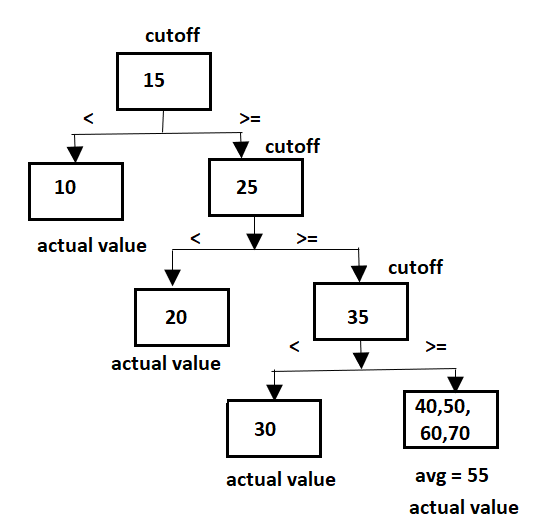# Day 52(ML) — Decision Tree (Handling of continuous data & Regression)Photo by Xavier L. on Unsplash

On Day50 we’ve started with a decision tree and discussed discrete predictors along with classification requirement. This article will continue with the algorithm but gives attention to continuous independent variables as well as the regression use case.

Table of contents:

• Input predictors are continuous for Classification
• Regression Requirement
• Feature Importance

Input predictors are continuous for Classification: As we already know, branching in a typical classification starts by computing impurity measures such as Entropy, Information gain and Gini index. Here also we calculate the same measures but instead of distinct categorical values, we will be using averages to decide the split as the input data is continuous numbers. Let’s consider the below data (i.e) based on the ‘Distance’ the target ‘Performance’ is computed.

Step1: Sort the data(ascending order)based on the continuous independent variable.

Step2: Take the average of two consecutive numbers.

Step3: For quantitative data, there will be only two splits based on the cutoff considered. Either ≤ or >. The check is applied to all the average values computed. Whichever threshold results in the lowest impurity, that will be considered as final. we’ll evaluate the Gini impurity for the data.

By looking at the split we can quickly conclude, ‘Tree4’ has the least impurity(zero). The rest of the split has a mix of both the target labels. The impurity calculations are similar to the ones we have already done for the discrete variable(as we have the counts now).

Regression Requirement: The regression results in numerical outcomes, so how do we start the branching and what is the criteria to decide the best split?. It can be thought of as binning for easy understanding. Say if we have 10 records, then we create 5 bins (each containing two records). The output of the bin is the average of all the values present. Then, the mean value is compared with the actual output to verify which one is giving the least mean squared error.Input & output both are continuous

For discrete input, the branching will be simple as it is based on the categorical values.

Feature Importance: We check feature by feature while performing the split at each step, this, in turn, provides information about significant predictors. The independent variables used in the top layers have a higher influence on the dependent target when compared to the ones in the bottom-most layer. By using the feature importance, we can also reduce the dimensionality by eliminating the input variables which have less predicting power.

Recommended videos:

https://www.youtube.com/watch?v=7VeUPuFGJHk&t=908s

https://www.youtube.com/watch?v=g9c66TUylZ4&t=322s

AI Enthusiast | Blogger✍

## More from Nandhini N

AI Enthusiast | Blogger✍